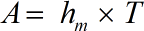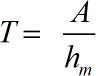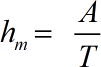Request a Tool

Mean Depth Calculator

Calculate the hydraulic mean depth of water using the sophisticated online Mean Depth Calculator.

Output

Area of Section Flow
0

Formula• A = Area of Section Flow
• hm = Mean Depth
• T = Top Water Surface Width

Defination / Uses

The average depth of water in a stream channel or conduit is referred to as mean depth. The cross-sectional area divided by the surface width equals the mean depth.

Output

Water Surface Width
0

Formula• T = Top Water Surface Width
• hm = Mean Depth
• A = Area of Section Flow

Defination / Uses

The average depth of water in a stream channel or conduit is referred to as mean depth. The cross-sectional area divided by the surface width equals the mean depth.

Mean Depth
0

Formula• hm = Mean Depth
• T = Top Water Surface Width
• A = Area of Section Flow

Defination / Uses

The average depth of water in a stream channel or conduit is referred to as mean depth. The cross-sectional area divided by the surface width equals the mean depth.

How to use mean depth calculator?

There are only two basic steps.

• First select the option from dropdown. What you need to calculate.
• Then add the other values in their corresponding fields.

Thats it! You will get your answer in less than a second. Use upper given formula in case of manual calculation.

The mean depth of any object can be calculated using our mean depth calculator. The mean depth is computed by assigning a depth value to each space based on how many spaces it is away from the initial space, adding these values together, then dividing by the number of spaces in the system minus one (the original space). You can calculate the average depth if you have numerous objects with a depth. When all of the items are analysed together, the average depth is calculated. The average is calculated by multiplying the total of all depths by the number of depths available. For quick calculation use our weetools. No sign-up, registration OR captcha is required to use this tool.